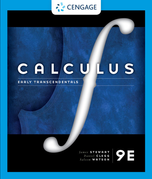×

×

Textbooks / Calculus / Calculus: Early Transcendentals 9

# Calculus: Early Transcendentals 9th Edition - Solutions by Chapter## Full solutions for Calculus: Early Transcendentals | 9th Edition

ISBN: 9781337613927Calculus: Early Transcendentals | 9th Edition - Solutions by Chapter

Solutions by Chapter
4 5 0 238 Reviews
##### ISBN: 9781337613927

Calculus: Early Transcendentals was written by Aimee Notetaker and is associated to the ISBN: 9781337613927. The full step-by-step solution to problem in Calculus: Early Transcendentals were answered by Aimee Notetaker, our top Calculus solution expert on 08/05/21, 04:08PM. Since problems from 132 chapters in Calculus: Early Transcendentals have been answered, more than 166582 students have viewed full step-by-step answer. This expansive textbook survival guide covers the following chapters: 132. This textbook survival guide was created for the textbook: Calculus: Early Transcendentals, edition: 9.

Key Calculus Terms and definitions covered in this textbook
• Algebraic model

An equation that relates variable quantities associated with phenomena being studied

• Circle graph

A circular graphical display of categorical data

• Circular functions

Trigonometric functions when applied to real numbers are circular functions

• Common logarithm

A logarithm with base 10.

• Conic section (or conic)

A curve obtained by intersecting a double-napped right circular cone with a plane

• End behavior asymptote of a rational function

A polynomial that the function approaches as.

• Hyperbola

A set of points in a plane, the absolute value of the difference of whose distances from two fixed points (the foci) is a constant.

• Implicitly defined function

A function that is a subset of a relation defined by an equation in x and y.

• Limaçon

A graph of a polar equation r = a b sin u or r = a b cos u with a > 0 b > 0

• Line graph

A graph of data in which consecutive data points are connected by line segments

• Linear correlation

A scatter plot with points clustered along a line. Correlation is positive if the slope is positive and negative if the slope is negative

• Open interval

An interval that does not include its endpoints.

• PH

The measure of acidity

• Plane in Cartesian space

The graph of Ax + By + Cz + D = 0, where A, B, and C are not all zero.

• Polar axis

See Polar coordinate system.

• Power regression

A procedure for fitting a curve y = a . x b to a set of data.

• Quotient rule of logarithms

logb a R S b = logb R - logb S, R > 0, S > 0

• Solve by substitution

Method for solving systems of linear equations.

• Stretch of factor c

A transformation of a graph obtained by multiplying all the x-coordinates (horizontal stretch) by the constant 1/c, or all of the y-coordinates (vertical stretch) of the points by a constant c, c, > 1.

• Window dimensions

The restrictions on x and y that specify a viewing window. See Viewing window.